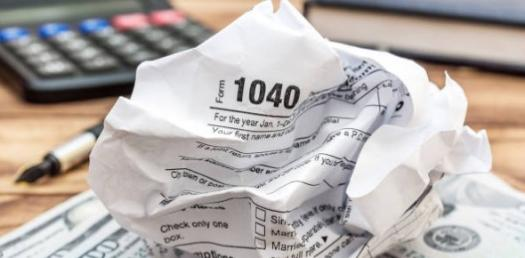# Accounting 302 Online Quizzes

6 Questions | Total Attempts: 305SettingsAccumulation of Styles' online quizzes

• 1.
What is the contract that gives rise to a bond?
• A.

Bond debenture

• B.

Bond Indenture

• 2.
The interest rate written in terms of the bond indenture is known as the?
• A.

Stated rate

• B.

Coupon rate

• C.

Nominal rate

• D.

Stated, coupon, or nominal rate

• 3.
What is the actual interest a bondholder receives?
• A.

Stated rate of interest

• B.

Interest yield

• C.

Effective yield

• 4.
How is the effective rate of interest calculated?
• A.

Multiply the carrying value of the bonds at the beginning of the period by the effective interest rate.

• B.

Multiply the face value of the bonds by the effective interest rate.

• 5.
What is common stock?
• A.

A residual corporate interest

• B.

Bears the ultimate risk of loss and receives the benefits of success

• C.

Generally control the management

• D.

Tend to profit most if the company is successful

• E.

All of the above

• 6.
What do preferred stock holders sacrifice?
• A.

A right to share in profits beyond the stated rate

• B.

Preemptive right

• C.

A right to a voice in management

• D.

A & C

Related TopicsBack to top